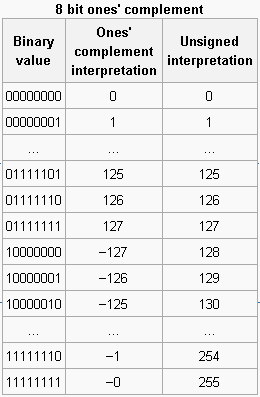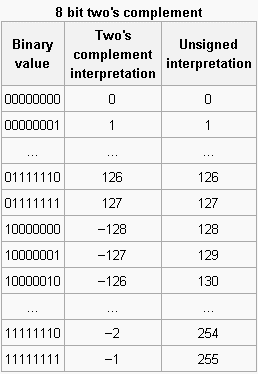# Signed number representations – 부호있는 수 표현

In mathematics, negative numbers in any base are represented in the usual way, by prefixing them with a “−” sign. However, on a computer, there are various ways of representing a number’s sign. This article deals with four methods of extending the binary numeral system to represent signed numbers: sign-and-magnitude, ones’ complement, two’s complement, and excess-N.

For most purposes, modern computers typically use the two’s-complement representation, but other representations are used in some circumstances.

Sign-and-magnitudeOne may first approach this problem of representing a number’s sign by allocating one sign bit to represent the sign: set that bit (often the most significant bit) to 0 for a positive number, and set to 1 for a negative number. The remaining bits in the number indicate the magnitude (or absolute value). Hence in a byte with only 7 bits (apart from the sign bit), the magnitude can range from 0000000 (0) to 1111111 (127). Thus you can represent numbers from −12710 to +12710 once you add the sign bit (the eighth bit). A consequence of this representation is that there are two ways to represent 0, 00000000 (0) and 10000000 (−0). Decimal −43 encoded in an eight-bit byte this way is 10101011.

This approach is directly comparable to the common way of showing a sign (placing a “+” or “−” next to the number’s magnitude). Some early binary computers (e.g. IBM 7090) used this representation, perhaps because of its natural relation to common usage. (Many decimal computers also used sign-and-magnitude.)

Ones’ complementAlternatively, a system known as ones’ complement can be used to represent negative numbers. The ones’ complement form of a negative binary number is the bitwise NOT applied to it — the complement of its positive counterpart. Like sign-and-magnitude representation, ones’ complement has two representations of 0: 00000000 (+0) and 11111111 (−0).

As an example, the ones’ complement form of 00101011 (43) becomes 11010100 (−43). The range of signed numbers using ones’ complement in a conventional eight-bit byte is −12710 to +12710.

To add two numbers represented in this system, one does a conventional binary addition, but it is then necessary to add any resulting carry back into the resulting sum. To see why this is necessary, consider the following example showing the case of the addition of −1 (11111110) to +2 (00000010).

In the previous example, the binary addition alone gives 00000000—not the correct answer! Only when the carry is added back in does the correct result (00000001) appear.

This numeric representation system was common in older computers; the PDP-1 and UNIVAC 1100/2200 series, among many others, used ones’-complement arithmetic.

(A remark on terminology: The system is referred to as “ones’ complement” because the negation of x is formed by subtracting x from a long string of ones. Two’s complement arithmetic, on the other hand, forms the negation of x by subtracting x from a single large power of two.)

The IPv4 header checksum uses ones’ complement arithmetic, here even on two’s complement machines the inconvenience of having to add back a carry is a desirable error-checking property, because “it is equally sensitive to errors in all bit positions”. In the UDP protocol one of the two representations of 0 provided by the ones’ complement arithmetic is used for indicating that the optional checksum feature has been omitted (all 0s), whereas the other (all 1s) indicates a checksumming result of 0.

Note that the ones’ complement representation of a negative number can be obtained from the sign-magnitude representation merely by bitwise complementing the magnitude.Two’s complement
(http://en.wikipedia.org/wiki/Twos_complement)
The problems of multiple representations of 0 and the need for the end-around carry are circumvented by a system called two’s complement. In two’s complement, negative numbers are represented by the bit pattern which is one greater (in an unsigned sense) than the ones’ complement of the positive value.

In two’s-complement, there is only one zero (00000000). Negating a number (whether negative or positive) is done by inverting all the bits and then adding 1 to that result. Addition of a pair of two’s-complement integers is the same as addition of a pair of unsigned numbers (except for detection of overflow, if that is done). For instance, a two’s-complement addition of 127 and −128 gives the same binary bit pattern as an unsigned addition of 127 and 128, as can be seen from the above table.

출처: 위키사전